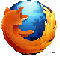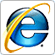# Arduino Digital Pins

There are 20 I/O pins on the Arduino Duemilanove. 14 pins are named digital pins and 6 pins are named analog pins. If necessary these analog pins can also be configured as I/O pins. The corresponding Arduino pin numbers to Atmega168 chip are as following.

### Atmega168 Pin

PIN0(RX) PD0(2)
PIN1(TX) PD1(3)
PIN2 PD2(4)
PIN3(PWM) PD3(5)
PIN4 PD4(6)
PIN5(PWM) PD5(11)
PIN6(PWM) PD6(12)
PIN7 PD7(13)
PIN8 PB0(14)
PIN9(PWM) PB1(15)
PIN10(PWM) PB2(16)
PIN11(PWM) PB3(17)
PIN12 PB4(18)
PIN13 PB5(19)
PIN14(ANALOG0) PC0(23)
PIN15(ANALOG1) PC1(24)
PIN16(ANALOG2) PC2(25)
PIN17(ANALOG3) PC3(26)
PIN18(ANALOG4) PC4(27)
PIN19(ANALOG5) PC5(28)

## Pin Mode

Pins can be configured as either input or output by command pinMode().

The syntax is pinMode(pin,mode), where pin is the pin number and mode is either "INPUT" or "OUTPUT". e.g.

pinMode(10,INPUT);    //Digital pin 10 is used as input

pinMode(11,OUTPUT);   //Digital pin 11 is used as output;

## Pin State

There are two states for a digital pin, for examples HIGH or LOW. However the meaning of state is depending on the pin mode.

For input mode, a HIGH state means a 3V or higher voltage signal is present at the pin, while a LOW state means a 2v or lower voltage signal is present at the pin.

For output mode, a HIGH state means a 5V signal is set at the pin, while a LOW state means a 0v signal is set at the pin.

## Pin Digital Input

The syntax is digitalRead(pin), where pin is the pin number. The return value is "HIGH" or "LOW". e.g.

pinMode(10,INPUT);    //digital pin 10 is used as input

int val=0;               //variable val to store the read value.

## Pin Digital Output

The state of output pin can be set by command digitalWrite()

The syntax is digitalWrite(pin,value), where pin is the pin number and value is "HIGH" or "LOW". e.g.

pinMode(11,OUTPUT);   //digital pin 11 is used as output;

digitalWrite(11,HIGH);    //sets digital pin 11 to HIGH state

## Pull-up Resistor

To prevent the floating of input pin state when the pin is not connected to anything, a pull-up or pull-down resistor can be added to keep an input to a known state. For the Atmega168, there are internal pull-up resistances and they can be selected for each pin. These built-in pull-up resistors can be activated as following:

pinMode(10,INPUT);    //use digital pin 10 as input

digitalWrite(10, HIGH);//activate internal pull-up resistance.

## Pin 13

Arduino Duemilanove has a build-in resistor and LED circuit soldered to Pin 13. When pin 13 is used as a digital input with a pull-up resistor, the LED will be lighted up and the pin voltage will hang at around 1.8V instead of 5V. Therefore, an external pull-down resistor should be used when using the pin 13 as a digital input.47n/a